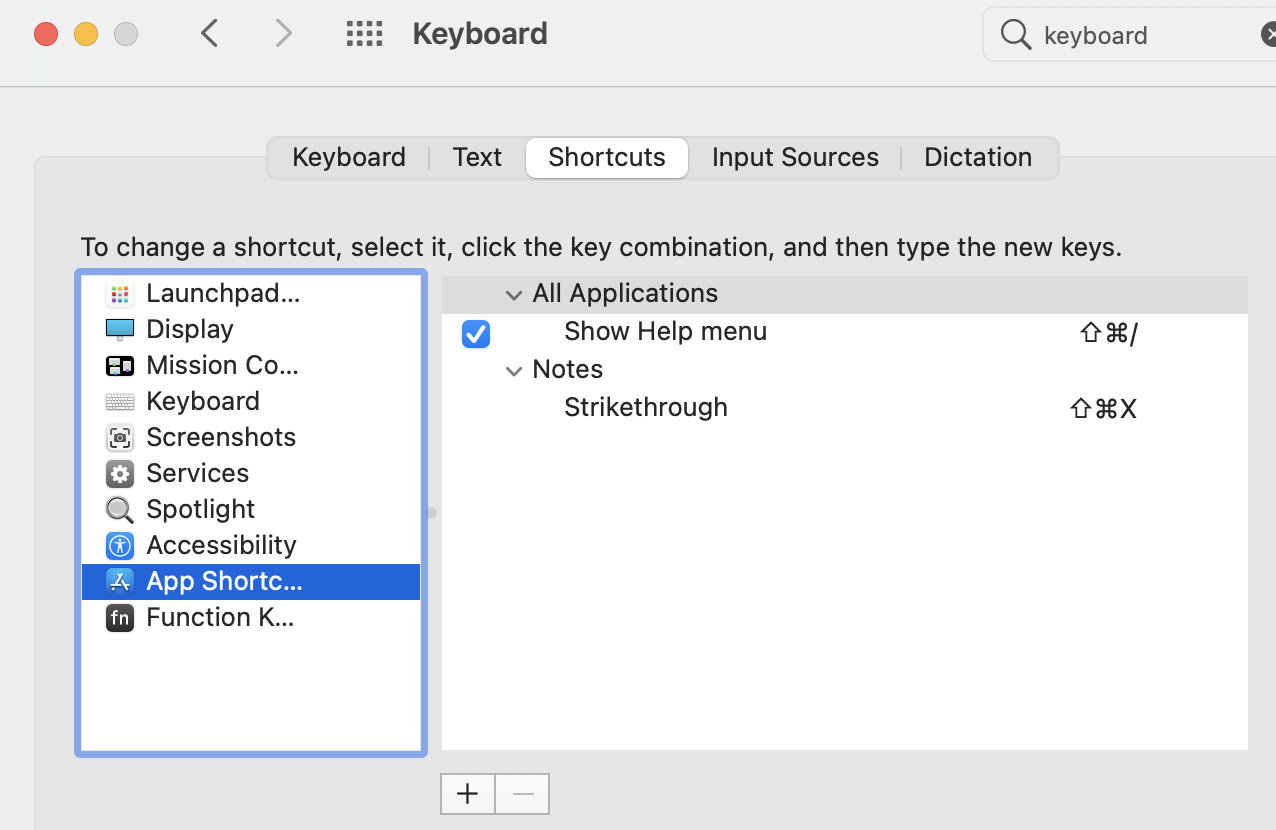### VBA Macro to copy Excel data into MS Word table

The below code will help you to copy the MS Excel data into MS Word. The main thing to note here is, the Excel data is copied into Word in a table format. This is a very basic VBA code, you can extend this to add other features.

To add this code to your excel, Open VBA editor in Excel or you can press F11. Insert a module, paste this code. Come back to excel by pressing F11 again. Under macros you can see the name of subroutine 'CopyFromExcelToWord' appearing, this is your macro.

Sub CopyFromExcelToWord()
Dim objWord
Dim objDoc
Dim objRange
Dim total_rows
Dim total_cols
Dim objTable
Dim tmp_row
Dim tmp_col
total_rows = Cells(1, 10).Value
total_cols = Cells(2, 10).Value
Set objWord = CreateObject("Word.Application")

Set objRange = objDoc.Range
objWord.Visible = True

'objWord.SaveAs ("C:\Users\sanjeeva\Documents\krishna\personal\videos\a.docm")
Set objTable = objDoc.Tables(1)
objTable.Borders.Enable = True
For tmp_row = 1 To total_rows
For tmp_col = 1 To total_cols
objTable.Cell(tmp_row, tmp_col).Range.Text = Cells(tmp_row, tmp_col)
Next
Next
End Sub